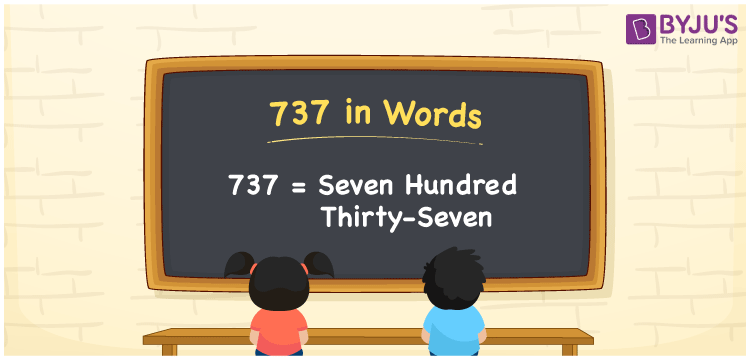# 737 in Words

737 in words is written as Seven hundred thirty-seven. In both the International System of Numerals and the Indian System of Numerals, 737 is written as Seven hundred thirty-seven. The number 737 is a Cardinal Number as it defines some quantity. For example, “there is a discount of 737 rupees”.

 737 in Words Seven hundred thirty-seven Seven hundred thirty-seven in Number 737

## 737 in English Words

We write 737 in English Words using the letters of English alphabets. Therefore, we read 737 in English as “Seven hundred thirty-seven”## How to Write 737 in Words?

To write 737 in words, we shall use the place value chart. In the place value chart, write 7 in the hundreds, 3 in the tens, and 7 in the ones, respectively. Now let us make a place value chart to write the number 737 in words.

 Hundreds Tens Ones 7 3 7

Thus, we can write the expanded form as

7 × Hundred + 3 × Ten + 7 × One

= 7 × 100 + 3 × 10 + 7 × 1

= 700 + 30 + 7

= 737

= Seven hundred thirty-seven.

737 is a natural number, the successor of 736 and the predecessor of 737.

737 in words – Seven hundred thirty-seven

• Is 737 an odd number? – Yes
• Is 737 an even number? – No
• Is 737 a perfect square number? – No
• Is 737 a perfect cube number? – No
• Is 737 a prime number? – No
• Is 737 a composite number? – Yes

## Frequently Asked Questions on 737 in Words

Q1

### How to write 737 in words?

737 in words is written as Seven hundred thirty-seven.
Q2

### How to write 737 in words in the International and Indian System of Numerals?

In both the system of numerals, 737 is written as Seven hundred thirty-seven.
Q3

### What is the successor of 737?

The successor of 737 is 738.